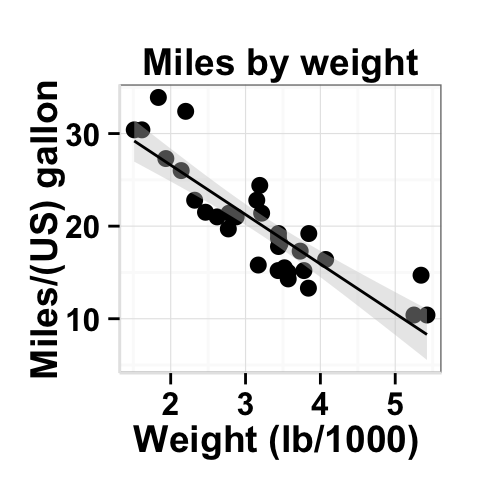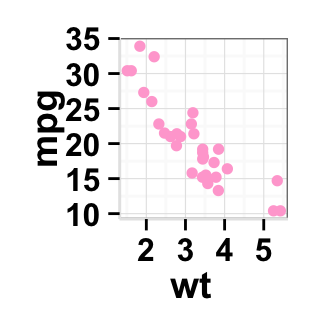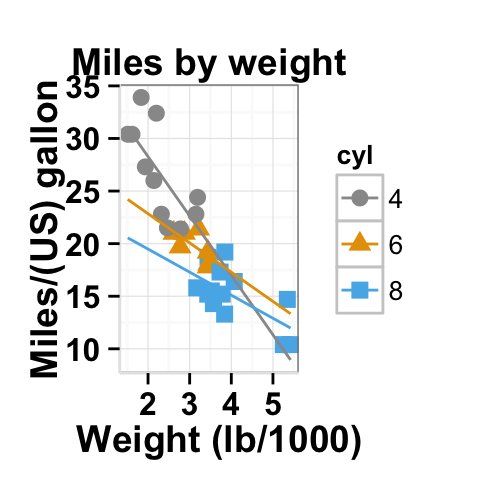# Introduction

ggplot2.scatterplot is an easy to use function to make and customize quickly a scatter plot using R software and ggplot2 package. ggplot2.scatterplot function is from easyGgplot2 R package. An R script is available in the next section to install the package.

The aim of this tutorial is to show you step by step, how to plot and customize a scatter plot using ggplot2.scatterplot function.

At the end of this tutorial you will be able to draw, with few R code, the following plots:ggplot2.scatterplot function is described in detail at the end of this document.

# Install and load easyGgplot2 package

easyGgplot2 R package can be installed as follow :

``````install.packages("devtools")
library(devtools)
install_github("easyGgplot2", "kassambara")``````

Load the package using this R code :

``library(easyGgplot2)``

# Data format

The data must be a data.frame (columns are variables and rows are observations).

`mtcars` data is used in the following examples.

``````df <- mtcars[, c("mpg", "cyl", "wt", "qsec", "vs")]
``````##                    mpg cyl    wt  qsec vs
## Mazda RX4         21.0   6 2.620 16.46  0
## Mazda RX4 Wag     21.0   6 2.875 17.02  0
## Datsun 710        22.8   4 2.320 18.61  1
## Hornet 4 Drive    21.4   6 3.215 19.44  1
## Hornet Sportabout 18.7   8 3.440 17.02  0
## Valiant           18.1   6 3.460 20.22  1``````

mtcars : Motor Trend Car Road Tests.

Description: The data comprises fuel consumption and 10 aspects of automobile design and performance for 32 automobiles (1973 - 74 models).

Format: A data frame with 32 observations on 4 variables.

• [, 1] mpg Miles/(US) gallon
• [, 2] cyl Number of cylinders
• [, 3] wt Weight (lb/1000)
• [, 4] qsec 1/4 mile time
• [, 5] vs V/S

# Basic scatter plot plot

``````# Basic scatter plot of mpg according to cyl
ggplot2.scatterplot(data=df, xName='wt',yName='mpg')
# Change point size
ggplot2.scatterplot(data=df, xName='wt',yName='mpg', size=3)
# change point size according to a numeric variable (qsec)
ggplot2.scatterplot(data=df, xName='wt',yName='mpg',
mapping=aes(size = qsec))``````## Scatterplot with regression line

``````#Add linear regression line
ggplot2.scatterplot(data=df, xName='wt',yName='mpg',

ggplot2.scatterplot(data=df, xName='wt',yName='mpg',

#Use loess (local fiting as smooth method)
ggplot2.scatterplot(data=df, xName='wt',yName='mpg',# Change the line type and the point shapes of the scatter plot

Different point shapes and line types can be used in the plot. By default, ggplot2 uses solid line type and circle shape.

``````# Change the scatter plot line type;
# change point shape, size and fill color
ggplot2.scatterplot(data=df, xName='wt',yName='mpg',
linetype="dashed", shape=23, size=3, fill="blue")                  ``````# Scatter plot plot with multiple groups

We will set color/shape by another variable (cyl)

``````# plot of variable 'mpg' according to xName 'wt'.
# The plot is colored by the groupName 'cyl'
ggplot2.scatterplot(data=df, xName='wt',yName='mpg',
groupName="cyl")
# Change group colors
ggplot2.scatterplot(data=df, xName='wt',yName='mpg',
groupName='cyl', size=3,
backgroundColor="white",
groupColors=c('#999999','#E69F00', '#56B4E9'))
# Use unique color for all groups
ggplot2.scatterplot(data=df, xName='wt',yName='mpg',
groupName='cyl', size=3,
backgroundColor="white", setColorByGroupName=FALSE)  ````````````# Add regression line and confidence interval
ggplot2.scatterplot(data=df, xName='wt',yName='mpg',
groupName='cyl', size=3, backgroundColor="white",
groupColors=c('#999999','#E69F00', '#56B4E9'),
# Extend the regression lines beyond the domain of the data
ggplot2.scatterplot(data=df, xName='wt',yName='mpg',
groupName='cyl', size=3, backgroundColor="white",
groupColors=c('#999999','#E69F00', '#56B4E9'),
# Set point shape by groupName
ggplot2.scatterplot(data=df, xName='wt',yName='mpg',
groupName='cyl', size=3, backgroundColor="white",
groupColors=c('#999999','#E69F00', '#56B4E9'),
setShapeByGroupName=TRUE) ``````## Parameters

The arguments that can be used to customize x and y axis are listed below :

Parameters Description
mainTitle the title of the plot
mainTitleFont a vector of length 3 indicating respectively the size, the style (“italic”, “bold”, “bold.italic”) and the color of x and y axis titles. Default value is: mainTitleFont=c(14, “bold”, “black”).
xShowTitle, yShowTitle if TRUE, x and y axis titles will be shown. Set the value to FALSE to hide axis labels. Default values are `TRUE`.
xtitle, ytitle x and y axis labels. Default values are `NULL`.
xtitleFont, ytitleFont a vector of length 3 indicating respectively the size, the style and the color of x and y axis titles. Possible values for the style:“plain”, “italic”, “bold”, “bold.italic”. Color can be specified as an hexadecimal code (e.g: “#FFCC00”) or by the name (e.g : “red”, “green”). Default values are `xtitleFont=c(14,"bold", "black"), ytitleFont=c(14,"bold", "black")`.
xlim, ylim limit for the x and y axis. Default values are `NULL`.
xScale, yScale x and y axis scales. Possible values : c(“none”, “log2”, “log10”). e.g: yScale=“log2”. Default values are `NULL`.
xShowTickLabel, yShowTickLabel if TRUE, x and y axis tick mark labels will be shown. Default values are `TRUE`.
xTickLabelFont, yTickLabelFont a vector of length 3 indicating respectively the size, the style and the color of x and y axis tick label fonts. Default value are `xTickLabelFont=c(12, "bold", "black"), yTickLabelFont=c(12, "bold", "black")`.
xtickLabelRotation, ytickLabelRotation Rotation angle of x and y axis tick labels. Default value are `0`.
hideAxisTicks if TRUE, x and y axis ticks are hidden. Default value is `FALSE`.
axisLine a vector of length 3 indicating respectively the size, the line type and the color of axis lines. Default value is `c(0.5, "solid", "#E5E5E5")`.

## Main title and axis labels

``````# Change main title and axis titles
ggplot2.scatterplot(data=df, xName='wt',yName='mpg',
mainTitle="Miles per gallon \n according to the weight",
xtitle="Weight (lb/1000)", ytitle="Miles/(US) gallon")
# Customize title styles. Possible values for the font style :
# 'plain', 'italic', 'bold', 'bold.italic'.
ggplot2.scatterplot(data=df, xName='wt',yName='mpg',
mainTitle="Miles per gallon \n according to the weight",
xtitle="Weight (lb/1000)", ytitle="Miles/(US) gallon",
mainTitleFont=c(14,"bold.italic", "red"),
xtitleFont=c(14,"bold", "#993333"),
ytitleFont=c(14,"bold", "#993333"))
# Hide x an y axis titles
ggplot2.scatterplot(data=df, xName='wt',yName='mpg',
xShowTitle=FALSE, yShowTitle=FALSE)               ``````## Axis ticks

``````# Axis ticks labels and orientaion
ggplot2.scatterplot(data=df, xName='wt',yName='mpg',
xShowTitle=FALSE, yShowTitle=FALSE,
xTickLabelFont=c(14,"bold", "#993333"),
yTickLabelFont=c(14,"bold", "#993333"),
xtickLabelRotation=45, ytickLabelRotation=45)
# Hide axis tick labels
ggplot2.scatterplot(data=df, xName='wt',yName='mpg',
xShowTitle=FALSE, yShowTitle=FALSE,
xShowTickLabel=FALSE, yShowTickLabel=FALSE)
# Hide axis ticks
ggplot2.scatterplot(data=df, xName='wt',yName='mpg',
xShowTitle=FALSE, yShowTitle=FALSE,
xShowTickLabel=FALSE, yShowTickLabel=FALSE,
hideAxisTicks=TRUE)
# AxisLine : a vector of length 3 indicating the size,
#the line type and the color of axis lines
ggplot2.scatterplot(data=df, xName='wt',yName='mpg',
axisLine=c(1, "solid", "darkblue"))``````## Background and colors

### Change scatter plot plot background and point colors

``````# change background color to "white". Default is "gray"
ggplot2.scatterplot(data=df, xName='wt',yName='mpg',
backgroundColor="white")
# Change background color to "lightblue" and grid color to "white"
ggplot2.scatterplot(data=df, xName='wt',yName='mpg',
backgroundColor="lightblue", gridColor="white")
# Change point color
ggplot2.scatterplot(data=df, xName='wt',yName='mpg',
# Remove grid; Remove Top and right border around the plot
ggplot2.scatterplot(data=df, xName='wt',yName='mpg',
removePanelGrid=TRUE,removePanelBorder=TRUE,
axisLine=c(0.5, "solid", "black"))``````### Change scatter plot color according to the group

Colors can be specified as a hexadecimal RGB triplet, such as `"#FFCC00"` or by names (e.g : `"red"` ). You can also use other color scales, such as ones taken from the RColorBrewer package. The different color systems available in R have been described in detail here.

To change scatter plot color according to the group, you have to specify the name of the data column containing the groups using the argument `groupName`. Use the argument `groupColors`, to specify colors by `hexadecimal` code or by `name`. In this case, the length of groupColors should be the same as the number of the groups. Use the argument `brewerPalette`, to specify colors using `RColorBrewer`palette.

``````# Change point color according to another variable
ggplot2.scatterplot(data=df, xName='wt',yName='mpg',
size=3, mapping=aes(colour = qsec))
# change point color transparency (alpha) according
# to another variable. alpha is the transparency degree of color.
#The value can variate from 0 (total transparency)
# to 1 (no transparency)
ggplot2.scatterplot(data=df, xName='wt',yName='mpg', size=3,
mapping=aes(alpha = qsec), color="darkgreen")
#Change point color according to a factor variable (group)
ggplot2.scatterplot(data=df, xName='wt',yName='mpg',
size=3, groupName="cyl")

#Change group colors using hexadecimal colors
ggplot2.scatterplot(data=df, xName='wt',yName='mpg',
groupName="cyl", size=3,
groupColors=c('#999999','#E69F00','#56B4E9'))

# Change group colors using brewer palette: "Paired"
ggplot2.scatterplot(data=df, xName='wt',yName='mpg', size=3,
groupName="cyl", brewerPalette="Paired")``````Color can also be changed by using names as follow :

``````# Change group colors using color names
ggplot2.scatterplot(data=df, xName='wt',yName='mpg', size=3,
groupName="cyl",
groupColors=c('aquamarine3','chartreuse1','goldenrod1'))``````

## Legend

### Legend position

``````# Change the legend position to "top"
# (possible values: "left","top", "right", "bottom")
ggplot2.scatterplot(data=df, xName='wt',yName='mpg',
groupName="cyl", legendPosition="top")
# legendPosition can be also a numeric vector c(x, y)
ggplot2.scatterplot(data=df, xName='wt',yName='mpg',
groupName="cyl", legendPosition=c(0.8,0.2))
# Remove plot legend
ggplot2.scatterplot(data=df, xName='wt',yName='mpg',
groupName="cyl", showLegend=FALSE)``````It is also possible to position the legend inside the plotting area. You have to indicate the x, y coordinates of legend box. x and y values must be between 0 and 1. c(0,0) corresponds to “bottom left” and c(1,1) corresponds to “top right” position.

### Legend background color, title and text font styles

``````# Change legend background color, title and text font styles
ggplot2.scatterplot(data=df, xName='wt',yName='mpg',
groupName="cyl",
#legendPosition=c("right", "left","top", "bottom")
legendPosition="right",
#legendTitleFont=c(size, style, color)
legendTitle="Dose (mg)", legendTitleFont=c(10, "bold", "blue"),
#legendTextFont=c(size, style, color)
legendTextFont=c(10, "bold.italic", "red"),
#legendBackground: c(fill, lineSize, lineType, lineColor)
legendBackground=c("lightblue", 0.5, "solid", "darkblue" )
)``````## Axis scales

Possible values for x axis scale are “none”, “log2” and log10. Default value is “none”.

``````# Change y axis limit
ggplot2.scatterplot(data=df, xName='wt',yName='mpg',
xlim=c(0,6) ,ylim=c(0,40))
# Log scale. yScale="log2".
# Possible value="none", "log2" and "log10"
#Default value is "none"
ggplot2.scatterplot(data=df, xName='wt',yName='mpg',
xScale="log2", yScale="log2")``````## Create a customized plots with few R code

``````#Customized scatterplot
ggplot2.scatterplot(data=df, xName='wt',yName='mpg', size=3,
backgroundColor="white", xtitle="Weight (lb/1000)",
ytitle="Miles/(US) gallon",
mainTitle="Miles per gallon \n according to the weight")
# Remove grid; Remove Top and right border around the plot
ggplot2.scatterplot(data=df, xName='wt',yName='mpg', size=3,
backgroundColor="white",
xtitle="Weight (lb/1000)", ytitle="Miles/(US) gallon",
mainTitle="Miles per gallon \n according to the weight",
removePanelGrid=TRUE,removePanelBorder=TRUE,
axisLine=c(0.5, "solid", "black"))

# Change point color according to the factor cyl
ggplot2.scatterplot(data=df, xName='wt',yName='mpg', size=3,
groupName="cyl",
groupColors=c('#999999','#E69F00','#56B4E9'),
backgroundColor="white",
xtitle="Weight (lb/1000)", ytitle="Miles/(US) gallon",
mainTitle="Miles by weight")``````# Faceting : split a plot into a matrix of pannels

The facet approach splits a plot into a matrix of panels. Each panel shows a different subset of the data.

## Facet with one variable

``````# Facet according to the supp variable
ggplot2.scatterplot(data=df, xName='wt', yName="mpg",
faceting=TRUE, facetingVarNames="cyl")
# Change the direction. possible values are "vertical", "horizontal".
# default is vertical.
ggplot2.scatterplot(data=df, xName='wt', yName="mpg",
faceting=TRUE, facetingVarNames="cyl",
facetingDirection="horizontal") ``````## Facet with two variables

``````# Facet by two variables: cyl and vs.
#Rows are cyl and columns are vs
ggplot2.scatterplot(data=df, xName='wt', yName="mpg",
faceting=TRUE, facetingVarNames=c("cyl", "vs"))
# Facet by two variables: reverse the order of the 2 variables
# Rows are vs and columns are cyl
ggplot2.scatterplot(data=df, xName='wt', yName="mpg",
faceting=TRUE, facetingVarNames=c("vs", "cyl"))``````## Facet scales

By default, all the panels have the same scale (`facetingScales="fixed"`). They can be made independent, by setting scales to `free`, `free_x`, or `free_y`.

``````#Facet with free scales
ggplot2.scatterplot(data=df, xName='wt', yName="mpg",
faceting=TRUE, facetingVarNames=c("vs", "cyl"),
facetingScales="free")``````As you can see in the above plot, y axis have different scales in the different panels.

## Facet label apperance

``````# Change facet text font. Possible values for the font style:
#'plain', 'italic', 'bold', 'bold.italic'.
ggplot2.scatterplot(data=df, xName='wt', yName="mpg",
faceting=TRUE, facetingVarNames=c("vs", "cyl"),
facetingFont=c(12, 'bold.italic', "red"))
# Change the apperance of the rectangle around facet label
ggplot2.scatterplot(data=df, xName='wt', yName="mpg",
faceting=TRUE, facetingVarNames=c("vs", "cyl"),
facetingRect=list(background="white", lineType="solid",
lineColor="black", lineSize=1.5)
)``````# ggplot2.scatterplot function

## Description

Plot easily a scatter plot using easyGgplot2 R package.

## usage

``````ggplot2.scatterplot(data, xName, yName, groupName=NULL,
smoothingMethod=c("lm", "glm", "gam", "loess", "rlm"),
confidenceIntervalFill="#C7C7C7",
setColorByGroupName=TRUE, setShapeByGroupName=FALSE,
groupColors=NULL, brewerPalette=NULL,...)``````

## Arguments

Arguments Descriptions
data Data frame. Columns are variables and rows are observations.
xName The name of column containing x variable (i.e groups).
yName The name of column containing y variable.
groupName The name of column containing group variable. This variable is used to color plot according to the group.
regLineColor Color of regression line. Default value is blue.
regLineSize Weight of regression line. Default value is 0.5.
smoothingMethod Smoothing method (function) to use, eg. lm, glm, gam, loess, rlm. For datasets with n < 1000 default is loess. For datasets with 1000 or more observations defaults to gam. lm for linear smooths, glm for generalized linear smooths, loess for local smooths, gam fits a generalized additive model.
addConfidenceInterval Display confidence interval around smooth? (FALSE by default).
confidenceLevel Level controlling confidence region. Default is 95%.
confidenceIntervalFill Fill color of confidence interval.
setColorByGroupName If TRUE, points are colored according the groups. Default value is TRUE.
setShapeByGroupName If TRUE, point shapes are different according to the group. Default value is FALSE.
groupColors Color of groups. groupColors should have the same length as groups.
brewerPalette This can be also used to indicate group colors. In this case the parameter groupColors should be NULL. e.g: brewerPalette=“Paired”.
…. Other parameters passed on to ggplot2.customize custom function or to geom_smooth and to geom_point functions from ggplot2 package.

The other arguments which can be used are described at this link : ggplot2 customize. They are used to customize the plot (axis, title, background, color, legend, ….) generated using ggplot2 or easyGgplot2 R package.

## Examples

``````df <- mtcars
ggplot2.scatterplot(data=df, xName='wt',yName='mpg', size=3,
mainTitle="Miles per gallon \n according to the weight",
xtitle="Weight (lb/1000)", ytitle="Miles/(US) gallon")
#Or use this
plot<-ggplot2.scatterplot(data=df, xName='wt',yName='mpg', size=3)
plot<-ggplot2.customize(plot,
mainTitle="Miles per gallon \n according to the weight",
xtitle="Weight (lb/1000)", ytitle="Miles/(US) gallon")
print(plot)``````

# Easy ggplot2 ebook

Note that an eBook is available on easyGgplot2 package here.

September 2014 : First edition.

Licence : This document is under creative commons licence (http://creativecommons.org/licenses/by-nc-sa/3.0/).

# Infos

This analysis was performed using R (ver. 3.1.0), easyGgplot2 (ver 1.0.0) and ggplot2 (ver 1.0.0).